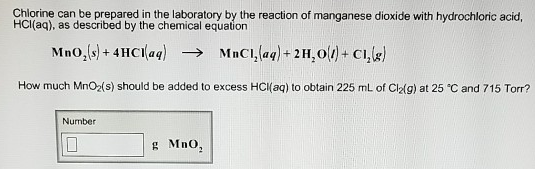# Problem: Chlorine can be prepared in the laboratory by the reaction of manganese dioxide with hydrochloric acid, HCl(aq), as described by the chemical equation MnO2 (s) + 4HCl (aq) → MnCl2 (aq) + 2 H2O (l) + Cl2 (g) How much MnO2(s) should be added to excess HCl(aq) to obtain 225 mL of Cl2(g) at 25° and 715 Torr?

###### FREE Expert Solution
91% (20 ratings)###### Problem Details

Chlorine can be prepared in the laboratory by the reaction of manganese dioxide with hydrochloric acid, HCl(aq), as described by the chemical equation

MnO(s) + 4HCl (aq) → MnCl2 (aq) + 2 H2O (l) + Cl2 (g)

How much MnO2(s) should be added to excess HCl(aq) to obtain 225 mL of Cl2(g) at 25° and 715 Torr?